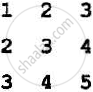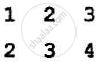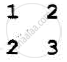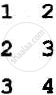Share

# Important Questions for CBSE (Science) Class 12 - CBSE - Computer Science (C++)

Subjects
Topics
Subjects
Popular subjects
Topics
Computer Science (C++)
< prev 1 to 20 of 96 next >

Write the type of C++ token (keywords) and the User-defined identifier from the following.

1) else

2) Long

3) 4Queue

4) _count

Appears in 1 question paper
Chapter:  Object Oriented Programming in C++
Concept: Concept of Object Oriented Programming in C++

The following C++ code during compilation reports errors as follows:

Error: 'ofstream' not declared

Error: 'strupr' not declared

Error: 'strcat' not declared

Error: 'FIN' not declared

Write the names of the correct header files, which must be included  to compile the code successfully

void main()
{
of stream FIN ("WISH. TXT") ;
char TEXT2[]="good day";
char TEXTl []="John! ";

strupr(TEXT2);
strcat(TEXTl , TEXT2);
FIN<<TEXTl<
Appears in 1 question paper
Chapter:  Object Oriented Programming in C++

Rewrite the following C++ code after removing any/all syntactical error with each correction underline

Note: Assume all required header files are already included in the program.

Typedef Count(int);
void main()
(
Count C;
cout<<"Enter the count:";
cin>>C;
for (K = 1 ; K<=C;K++)
cout<< C "*" K <<end1;
}
Appears in 1 question paper
Chapter:  Object Oriented Programming in C++
Concept: Concept of Object Oriented Programming in C++

Find and write the output of the following C++ program code:

Note: Assume all required header files are already included in the program

void Revert(int &Num, int Last=2)
{
Last =(Last%2==0)?Last+l: Last - l;
for(int C=l; C <= Last; C++)
Num+=C;
)

void main()
{
int A=20, B=4;
Revert(A, B) ;
cout<<A<<"&"<<B<<end1;
B--;
Revert{A,B);
cout<<A<<"#"<<B<<endl;
Revert{B);
cout<<A<<"#"<<B<<endl;
}
Appears in 1 question paper
Chapter:  Object Oriented Programming in C++
Concept: Member of a Class - Data Members and Member Functions (Methods)

Look at the following C++ code and find the possible output from the options (i) to (iv) following it. Also, write the highest and lowest values that can be assigned to the array A.

Note:

• Assume all the required header files are already being included in the code
• The function random(n) generates an integer between 0 and -1
void main()
{
randomize();
int A(4], C;
for(C=0; C<4; C++)
A(C] = random(C+l) + 10;
for (C=3; C >= 0; c--)
cout<<A(C]<<"@";
}

1) 13@10@11@10@

2) 15$14$12$10$

3) 12@11@13@10@

4) 12@11@10@10@

Appears in 1 question paper
Chapter:  Object Oriented Programming in C++
Concept: Pointers and Arrays - Array of Pointers, Pointer to an Array (1 Dimensional Array), Function Returning a Pointer, Reference Variables and Use of Alias

Which function(s) out of the following can be considered as an overloaded function(s) in the same program? Also, write the reason for not considering the other(s) as an overloaded function(s)

void Execute(char A,int B);    // Function 1

void Execute(int A,char B);    // Function 2

void Execute(int P=10) ;       // Function 3

void Execute();                    // Function 4

int Execute(int A);               // Function 5

void Execute(int &K);          // Function 6

Appears in 1 question paper
Chapter:  Object Oriented Programming in C++

Observe the following C++ code and answer the questions (i) and (ii).

Note: Assume all necessary files are included.

class FIRST
{
int Numl;
public:
void Display()
{
cout<<Numl<<endl;            //Member Function 1
}
};

class SECOND: public FIRST
{
int Num2;
public:
void Display()                //Member Function 2
{
cout<<Num2<<endl;
}
};

Void main()
{
SECOND S;
___________                    // Statement 1
___________                    // Statement 2

}

1) Which Objected Programming features is illustrated by the definition of classes FIRST and SECOND?

2) Write Statement 1 and Statement 2 to execute Member Function 1 and Member Function 2 respectively using the object S

Appears in 1 question paper
Chapter:  Object Oriented Programming in C++
Concept: Member of a Class - Data Members and Member Functions (Methods)

Write the definition of a class CONTAINER in C++ with the following description.

Private Members

- Type                  // int (1 for Cone, 2 for Cylinder)

- Volume              // float

- CalVolume()       // Member function to calculate

// volume as per the Type

Public Members

- GetValues ()    //A function to allow a user to enter of Radius, Height and Type. Also, call function CalVolume() from it.

- ShowAll ()      // A function to display Radius, Height, Type and Volume of Container

Appears in 1 question paper
Chapter:  Object Oriented Programming in C++
Concept: Member of a Class - Data Members and Member Functions (Methods)

Answer the question (i)  to (iv) based on the following:

class Teacher
{
int TCode;
protected:
char Name;
public:
Teacher();
void Enter() ; void Show();
};

class Course
(
int ID;
protected:
Char Title;
public:
Course();
void Initiate();
void Display();
};

class Schedule : public Course, private Teacher
{
int DD, MM, YYYY;
public:
Schedule();
void Start();
void View();
};

void main()
{
Schedule S;
}

1) Which type of Inheritance out of the following is illustrated in the above example?

2) Write the name of all the member which are directly accessible by the member function View() of Class Schedule.

3) Write the names of all the members, which are directly accessible by the object S of Class Schedule declared in the main() function.

4) What will be the order of execution of the constructors, when the object S of the Class Schedule Schedule is declared inside the main() function?

Appears in 1 question paper
Chapter:  Object Oriented Programming in C++
Concept: Inheritance in C++

A text file named MATTER.TXT contains some text which needs to be displayed such that every next character is separated by a symbol '#'.

Write a function definition for HashDisplay() in C++ that would display the entire content of the file MATTER.TXT in the desired format:

Example:

if the file Matter.TXT has the following content stored in it:

THE WORLD IS ROUND

The function HashDisplay() should display the following content:

T#H#E #W#O#R#L#D# #I#S# #R#O#U#N#D#

Appears in 1 question paper
Chapter:  Object Oriented Programming in C++
Concept: Implementation of Basic File Operations on Text and Binary File in C++

Write a definition for a function TotalTeachers( ) in C++ to read each object of a binary file SCHOOLS.DAT, find the total number teachers, whose data is stored in the file and display the same. Assume that the file SCHOOLS.DAT is created with the help objects of class SCHOOLS, which is defined below :

class SCHOOLS
{
int SCode;        //School Code
char SName;   //School Name
int NOT;          // Name of Teachers in the school

public:
void Display()
{
cout<<SCode<<"#"<<SName<<"#" << NOT << endl;
int RNOT() {return NOT;}
};
Appears in 1 question paper
Chapter:  Object Oriented Programming in C++
Concept: Implementation of Basic File Operations on Text and Binary File in C++

Find the output of the following C++ code considering that the binary file SCHOOL.DAT exists on the hard disk with the following records of 10 schools of the class SCHOOLS as declared in the previous question(4 b)

 SCode SName NOT 1001 Brains School 100 1003 Child Life School 115 1002 Care Share School 300 1006 Educa t for Life School 50 1005 Guru Shiahya Sadan 195 1004 Holy Education School 140 1010 Play School 95 1008 Innovate Excel School 300 1011 Premier Education School 200 1012 Uplifted Minds School 100
void main()
{
fstream SFIN;
SFIN.open("SCHOOLS.DAT", ios::binary|ios::in) ;
SCHOOLS S;
SFIN.seekg(S*sizeof(S));
S.Display();
cout<<"Record : "<<SFIN.tellg()/sizeof(S) + l <<endl;
SFIN.close();
}
Appears in 1 question paper
Chapter:  Object Oriented Programming in C++
Concept: Implementation of Basic File Operations on Text and Binary File in C++

Write the type of C++ tokens (keywords and user-defined identifiers) from the following:

1) new

2) While

3) case

4) Num_2

Appears in 1 question paper
Chapter:  Object Oriented Programming in C++
Concept: Concept of Object Oriented Programming in C++

Anil typed the following C++ code and during compilation, he found three errors as follows :

1) Function strlen should have a prototype

2) Undefined symbol cout

3) Undefined symbol endl

On asking, his teacher told him to include necessary header files in the code. Write the names of the header files, which Anil needs to include, for successful compilation and execution of the following code:

void main()
{
char Txt[] = "Welcome";
for(int C= 0; C<strlen(Txt); C++)
Txt[C] = Txt[C]+ 1;
cout<<Txt<<endl;
}
Appears in 1 question paper
Chapter:  Object Oriented Programming in C++

Rewrite the following C++ code after removing any/all syntactical errors with each correction underlined.

Note: Assume all required header files are already being included in the program.

void main()
{
cout<<"Enter an Alphabet:" ;
cin>>CB ;
switch(CH)
case 'A' · cout<<"Ant"; Break ;
case 'B' · cout<<"Bear"; Break;
}
Appears in 1 question paper
Chapter:  Object Oriented Programming in C++
Concept: Concept of Object Oriented Programming in C++

Find and write the output of the. following C++ program code

Note : Assume au· required header files are already included in the program.

#define Diff(N1,N2) ((N1>N2)?Nl-N2:N2-N1)
void main()
{
int A,B,NUM[] = {10,23,14,54,32};
for(int CNT =4; CNT > 0; CNT--)
{
A=NUM[CNT];
B=NUM[CNT-1];
cout<<Diff(A,B)<< '#';
}
}
Appears in 1 question paper
Chapter:  Object Oriented Programming in C++
Concept: Advantages of Object Oriented Programming Over Earlier Programming Methodologies in C++

Find and write the output of the following C++ program code :
Note : Assume all required header files are already being included in the program.

void main()
{
int *Point, Score[]={100,95,150 , 75 , 65, 120};
Point = Score;
for(int L = 0; L<6; L++)
{
if((*Poi nt)%10==0)
*Point /= 2;
else
*Point -= 2;
if((*Point) %5==0)
*Point /= 5;
Point++;
}
for(int L = 5; L>=O; L--)
cout<<Score[L]<<"*";
}
Appears in 1 question paper
Chapter:  Object Oriented Programming in C++
Concept: Pointers and Arrays - Array of Pointers, Pointer to an Array (1 Dimensional Array), Function Returning a Pointer, Reference Variables and Use of Alias

Look at the following C++ code and find the possible output(s) from the options (i) to (iv) following it. Also, write the maximum values that can be assigned to each of the variables N and M

Note:

• Assume all the .required header files are already being included in the code.
• The function random(n) generates an integer between 0 and n-1
void main()
{
randomize() ;
int N=random(3), M=random(4);
int DOCK = {{1,2,3},{2,3,4}, {3,4,5}};
for(int R=O; R<N; R++)
{
for(int C = 0; C< M; C++)
·        cout<<DOCK[R][C]<<" ";
cout<<endl;
}
}

 1)2)3)4)Appears in 1 question paper
Chapter:  Object Oriented Programming in C++
Concept: Member of a Class - Data Members and Member Functions (Methods)

Differentiate between protected .and private me~bers of a class. in context of Object Oriented Programming. Also, give a suitable example illustrating accessibility/non-accessibility of each using a class and an object in C++.

Appears in 1 question paper
Chapter:  Object Oriented Programming in C++
Concept: Using Private and Public Visibility Modes, Default Visibility Mode (Private)

Observe the following C++ code and answer the questions (i) and (ii).

Note: Assume all necessary files are included

class TEST
{
long TCode ;
char TTitle;
float Score;
public:
TEST()      //Member Function 1
{
TCode=100;strcpy(TTit1e,"FIRST Test"); Score=O;
};
TEST(TEST &T)    //Member Function 2
{
TCode=E.TCode+1;
strcpy(TTitle , T.TTitle);
Score=T.Score;
}
};

void main()
{
__________    //Statement 1
__________    //Statement 2
}

1) Which Object-Oriented Programming feature is illustrated by the Member Function 1 and the Member Function 2 together in the class TEST?

2) Write Statement 1 and Statement 2 to execute Member Function 1 and Member Function 2 respectively.

Appears in 1 question paper
Chapter:  Object Oriented Programming in C++
Concept: Member of a Class - Data Members and Member Functions (Methods)
< prev 1 to 20 of 96 next >